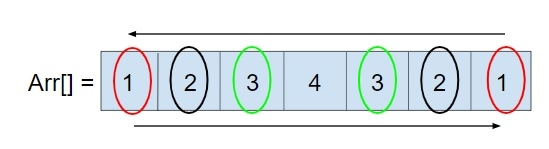# C Program to check if an Array is Palindrome or not

Given an array arr[] of any size n, our task is to find out that the array is palindrome or not. Palindrome is a sequence which can be read backwards and forward as same, like: MADAM, NAMAN, etc.

So to check an array is palindrome or not so we can traverse an array from back and forward like −## Example

Input: arr[] = {1, 0, 0, 1}
Output: Array is palindrome
Input: arr[] = {1, 2, 3, 4, 5}
Output: Array is not palindrome

Approach used below is as follows

We will traverse the array from starting as well as from the end until they both are equal and check whether the element from starting is same as the element from the end, then the array is palindrome else the array is not a palindrome.

## Algorithm

Start
In function int pallindrome(int arr[], int n)
Step 1-> initialize i, j, flag and assign flag as 0
Step 2-> Loop For i = 0, j=n-1 and i< n/2, j>=n/2 and i++, j--
If arr[i]!=arr[j] then,
Set flag as 1
Break
End If
End Loop
Step 3-> If flag == 1 then,
Return 0
Step 4-> Else
Return 1
End function
In function int main(int argc, char const *argv[])
Step 1-> Declare and initialize arr[] as {1, 0, 2, 3, 2, 2, 1}
Step 2-> Declare and initialize n as sizeof(arr)/sizeof(arr)
Step 3-> If pallindrome(arr, n) then,
Print "Array is pallindrome "
End if
Step 4-> Else
Print "Array is not pallindrome "
Return 0
End main
Stop

## Example

Live Demo

#include <stdio.h>
int pallindrome(int arr[], int n) {
int i, j, flag = 0;
for(i = 0, j=n-1; i< n/2, j>=n/2; i++, j--) {
if(arr[i]!=arr[j]) {
flag = 1;
break;
}
}
if (flag == 1)
return 0;
else
return 1;
}
int main(int argc, char const *argv[]) {
int arr[] = {1, 0, 2, 3, 2, 2, 1};
int n = sizeof(arr)/sizeof(arr);
if(pallindrome(arr, n)) {
printf("Array is pallindrome");
}
else
printf("Array is not pallindrome");
return 0;
}

## Output

If run the above code it will generate the following output −

Array is not palindrome